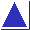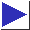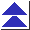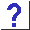# comp.lang.c FAQ list ·Question 20.1

Q: How can I return multiple values from a function?

A: There are several ways of doing this. (These examples show hypothetical polar-to-rectangular coordinate conversion functions, which must return both an x and a y coordinate.)

1. Pass pointers to several locations which the function can fill in:
```#include <math.h>

polar_to_rectangular(double rho, double theta,
double *xp, double *yp)
{
*xp = rho * cos(theta);
*yp = rho * sin(theta);
}

...

double x, y;
polar_to_rectangular(1., 3.14, &x, &y);
```
2. Have the function return a structure containing the desired values:
```struct xycoord { double x, y; };

struct xycoord
polar_to_rectangular(double rho, double theta)
{
struct xycoord ret;
ret.x = rho * cos(theta);
ret.y = rho * sin(theta);
return ret;
}

...

struct xycoord c = polar_to_rectangular(1., 3.14);
```
3. Use a hybrid: have the function accept a pointer to a structure, which it fills in:
```polar_to_rectangular(double rho, double theta,
struct xycoord *cp)
{
cp->x = rho * cos(theta);
cp->y = rho * sin(theta);
}

...

struct xycoord c;
polar_to_rectangular(1., 3.14, &c);
```
(Another example of this technique is the Unix system call stat.)
4. In a pinch, you could theoretically use global variables (though this is rarely a good idea).